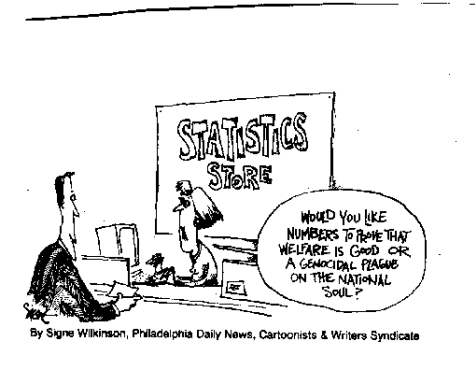## Data mining bias, a mathematical look. Part 1.

On this blog we have discussed the topic of data mining bias (DMB) in depth. I have sought to understand the way in which data mining bias works and how it changes depending on the particular process being tested. Today I am going to offer a mathematical view of DMB (from a frequentist’s approach) with which I intend to answer basic questions relevant to the nature and calculation of DMB. We will first go through the mathematical steps that yield useful equations to determine DMB and we will then see what happens with these equations as the logic space where we are mining for systems is changed. With this information we’ll be able to demonstrate some important mathematical properties of DMB that depend entirely on the properties of the space being explored.Let us assume that we have a logic space that contains all possible trading strategies that could ever be generated, a logic space that contains all possible systems that can exist within a financial time series. There are some trading systems that can be found within this time series that are interesting to us because of their historical performance characteristics (I call them S_real), these systems are in essence all systems that can be found due to spurious relationships within the data plus all systems that can be found due to real relationships within the data. By “real relationships” I mean relationships that are due to a true causal relationships between some past event and some future event while spurious relationships are simply systems that give a result due to the random arrangement of data. The whole exercise we carry out when we do DMB analysis is to determine the probability that a system from this group of interesting systems in the real data is the result of spurious relationships.

$P_{real}= \frac{S_{real}}{N}$ (Equation 1)

$P_{random_{i}}= \frac{S_{random_{i}}}{N}$ (Equation 2)

$P_{random}= \frac{\sum_{i=1}^T\frac{S_{random_{i}}}{N} }{T}$ (Equation 3)

$DMB = \frac{P_{random}}{P_{real}}$ (Equation 4)

There are several approaches to do this, the one I like the most involves building a probability distribution using random data sets. If we suppose that the probability to find systems that are interesting to us within the real data is given by equation 1 (where P_real is the probability, S_real are the number of systems that interest us and N are the total number of possible systems) and the probability to find systems that interest us on a random data set is given by equation 2, then we can calculate DMB by using equation 4, where we calculate the probability that spurious relationships (the average probability to find systems on random data sets as showed by equation 3) are found within our systems mined on the real financial time series. Equation three calculates the average probability to find a system that interests us within random time series by summing all probabilities for all our available random series (T) and then dividing them by T.

$DMB= \frac{\sum_{i=1}^T{S_{random_{i}}}}{T\times S_{real}}$ (Equation 5)

Equation 4 can be further simplified by using algebra to equation 5 which shows that the DMB is in essence dependent on the number of random data sets used, the total number of systems found on all random data sets and the number of systems found on the real data. As the number of systems found on real data increases then the probability that they are derived from spurious relationships becomes lower. If the number of systems found on random data increases then the probability that systems on the real data are due to spurious relationships also increases. It is also interesting to note here that the DMB does not depend on the number of systems in the logic space N, so in essence DMB is independent on the size of the space that is being used because the comparison between mining on real and random data is done over the same space, therefore completely negating the effect that the space has over the calculation of the DMB.

However this does not mean that the DMB cannot be underestimated by the choices of space being made. An important approximation in the calculation of DMB comes from the fact that we are not mining over the set of all available systems but we are mining on a smaller set. On my next post we are going to look at the consequences of reducing the space from the largest possible space containing all systems to a space that is a smaller set (what we really do when we mine systems). The way in which this reduction is made has important consequences in the accuracy of our DMB determination and you will see that we can mathematically show what happens in different cases.

Another critical aspect in the DMB evaluation is the construction of the random data. Our process assumes that the random data is in all ways identical to the real data except that no real causal relationships exist between past and future bars. This means that the real data has been shuffled or recreated in some manner in order to eliminate all these relationships while preserving statistical characteristics such as the distribution of returns and the distribution of ranges within the data. There are several ways in which this can be done and each one has different consequences in the number of systems that are derived from the random data. We will also explore this in future posts.

If you would like to learn more about data-mining bias and how we carry out the above analysis in practice using a cloud mining process based on the power of GPUs please consider joining Asirikuy.com, a website filled with educational videos, trading systems, development and a sound, honest and transparent approach towards automated trading.

You can leave a response, or trackback from your own site.